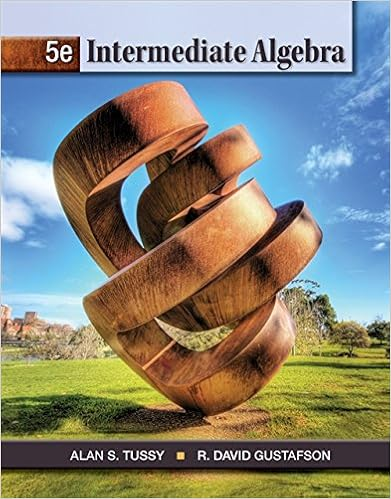# Compute the volume of the solid formed by revolving

• Homework Help
• 4
• 86% (7) 6 out of 7 people found this document helpful

This preview shows page 2 - 4 out of 4 pages.

##### We have textbook solutions for you!
The document you are viewing contains questions related to this textbook.The document you are viewing contains questions related to this textbook.
Chapter 1 / Exercise 36
Intermediate Algebra
Tussy/GustafsonExpert Verified
20.Compute the volume of the solid formed by revolving the region bounded by 2yxand2xyabout (a) the y-axis; (b) x= 1.26.Let Rbe the region bounded by 2yxand y= 4. Compute the volume of the solid formed by revolving Rabout the given line.(a)y= 4(b) the y-axis(c) y= 6(d) y= –2(e) x= 2(f) x= –4
32.Suppose that the circle 221xyis revolved about the y-axis. Show that the volume of the resulting solid is .43Section 5.34.Sketch the region, draw in a typical shell, identify the radius and height of the shell, andcompute the volume for the region bounded by ,,yx yx and 1,xrevolved about1x.6.Sketch the region, draw in a typical shell, identify the radius and height of the shell, andcompute the volume for the region bounded by 2yxand 0,11yx , revolved about x= 2.WA 1, p. 2
##### We have textbook solutions for you!
The document you are viewing contains questions related to this textbook.The document you are viewing contains questions related to this textbook.
Chapter 1 / Exercise 36
Intermediate Algebra
Tussy/GustafsonExpert Verified
8.Sketch the region, draw in a typical shell, identify the radius and height of the shell, andcompute the volume for the region bounded by 224xy, revolved about y= 412.Use cylindrical shells to compute the volume of the region bounded by 2xyand x= 4revolved about y= 222.Use the best method available to find the volume of the region bounded by22,(0)yxyx xand the y-axis revolved about (a) the ., .
x=
•••